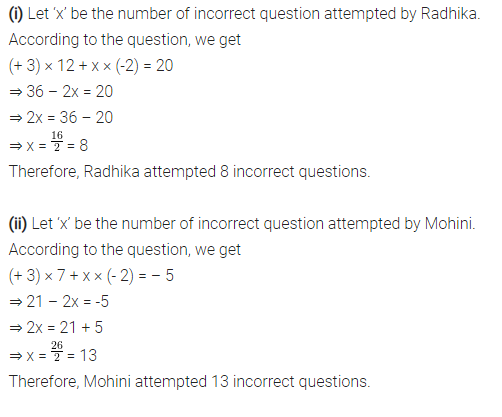# In a class test (+3) marks are given for every correct answer and (- 2) marks

In a class test (+3) marks are given for every correct answer and (- 2) marks are given for every incorrect answer and no marks for not attempting any question.
(i) Radhika scored 20 marks. If she has got 12 correct answers, how many questions has she attempted incorrectly?
(ii) Mohini scores – 5 marks in this test, though she has got 7 correct answers. How many questions has she attempted incorrectly ?Marks for correct answer = 3
Marks for incorrect answer = (-2)
Number of correct answer = 12

a = number of correct answer

12 X 3 + (-2) x a = 20
36 + (-a) = 20
36 - 20 = (-a)
36 - 20 = 16

Therefore number of incorrect answer are = 16

~The Brain…